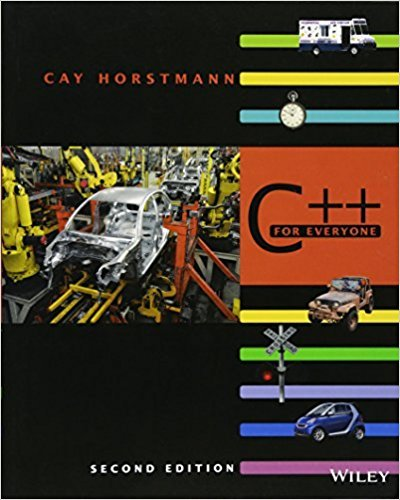×
Get Full Access to C++ For Everyone - 2 Edition - Chapter 7 - Problem R7.18
Get Full Access to C++ For Everyone - 2 Edition - Chapter 7 - Problem R7.18

×

# Which of the following assignments are legal in C++void f(int p[]){ int* q; const int*ISBN: 9780470927137 356

## Solution for problem R7.18 Chapter 7

C++ for Everyone | 2nd Edition

• Textbook Solutions
• 2901 Step-by-step solutions solved by professors and subject experts
• Get 24/7 help from StudySoup virtual teaching assistantsC++ for Everyone | 2nd Edition

4 5 1 308 Reviews
10
2
Problem R7.18

Which of the following assignments are legal in C++?void f(int p[]){ int* q; const int* r; int s; p = q; 1 p = r; 2 p = s; 3 q = p; 4 q = r; 5 q = s; 6 r = p; 7r = q; 8 r = s; 9 s = p; 10 s = q; 11 s = r; 12}

Step-by-Step Solution:
Step 1 of 3

ENGR 121 B Lecture Notes for 10/17/2016 Spencer Kociba ● Quiz on vector operations ● Truth tables ○ Both variables must be true (logical output) X Y xandy 0 0 0 0 1 0 1 0 0 1 1 1 ○ At least one must be true X Y xory 0 0 0 0 1 1 1 0 1 1 1 1 ○ Only 1 is true X Y x+y 0 0 0 0 1 1 1 0 1 1 1 0 ● If/else statements ○ Nested ifelse command ■ If condition 1 ● Action 2 ■ Elseif condition 2 ■ Elseif condition 3 ● Action 3 ■ (etc) ■ Else ● Action final ■ End

Step 2 of 3

Step 3 of 3

##### ISBN: 9780470927137

This textbook survival guide was created for the textbook: C++ for Everyone , edition: 2. C++ for Everyone was written by and is associated to the ISBN: 9780470927137. The full step-by-step solution to problem: R7.18 from chapter: 7 was answered by , our top Engineering and Tech solution expert on 03/08/18, 08:39PM. This full solution covers the following key subjects: . This expansive textbook survival guide covers 10 chapters, and 515 solutions. Since the solution to R7.18 from 7 chapter was answered, more than 269 students have viewed the full step-by-step answer. The answer to “Which of the following assignments are legal in C++?void f(int p[]){ int* q; const int* r; int s; p = q; 1 p = r; 2 p = s; 3 q = p; 4 q = r; 5 q = s; 6 r = p; 7r = q; 8 r = s; 9 s = p; 10 s = q; 11 s = r; 12}” is broken down into a number of easy to follow steps, and 65 words.

## Discover and learn what students are asking

Calculus: Early Transcendental Functions : Functions of Several Variables
?In Exercises 15 - 22, find all first partial derivatives. $$f(x, y)=4 x^{2}-2 x y+y^{2}$$

Calculus: Early Transcendental Functions : Space Coordinates and Vectors in Space
?In Exercises 1-4, plot the points in the same three-dimensional coordinate system. (a) (2, 1, 3) (b) (-1, 2, 1)

Statistics: Informed Decisions Using Data : Inference about the Difference between Two Medians: Dependent Samples
?In Problems 3–10, use the Wilcoxon matched-pairs signedranks test to test the given hypotheses at the a = 0.05 level of significance. The dependent sa

Unlock Textbook Solution